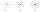# Lie/do not lie

The function is given by the rule f(x) = 8x+16. Determine whether point D[-1; 8] lies on this function. Solve graphically or numerically and give reasons for the your answer.

Result

#### Solution:Leave us a comment of example and its solution (i.e. if it is still somewhat unclear...):

Showing 0 comments:Be the first to comment!#### To solve this example are needed these knowledge from mathematics:

For Basic calculations in analytic geometry is helpful line slope calculator. From coordinates of two points in the plane it calculate slope, normal and parametric line equation(s), slope, directional angle, direction vector, the length of segment, intersections the coordinate axes etc.

## Next similar examples:

1. ShotsDetermine the percentage rate of keeper interventions if from 32 shots doesn't caught four shots.
2. Slope formFind the equation of a line given the point X(8, 1) and slope -2.8. Arrange your answer in the form y = ax + b, where a, b are the constants.
3. SlopeCalculate the slope of a line that intersects points (-84,41) and (-76,-32).
4. Days2minutesWrite a formula that converts x days to y minutes. The formula is:
5. Functions f,gFind g(1) if g(x) = 3x - x2 Find f(5) if f(x) = x + 1/2
6. CancerOf the 80 people 50 people ill cancer. What percentage of people isn't ill?
7. EnrollmentThe enrollment at a local college increased 4% over last year's enrollment of 8548. Find the increase in enrollment (x1) and the current enrollment (x2).
8. Sales offGoods is worth € 70 and the price of goods fell two weeks in a row by 10%. How many % decreased overall?
9. Percentages52 is what percent of 93?
10. Parcel 4To send a parcel by messenger within city limits costs 60 cents for the first pound and 48 cents for each additional pound. What is the cost, in cents, of sending a parcel weighing p=4 pounds?
11. Divisible by 5How many three-digit odd numbers divisible by 5, which are in place ten's number 3?
12. SlopeFind the slope of the line: x=t and y=1+t.
13. CandyPeter had a sachet of candy. He wanted to share with his friends. If he gave them 30 candies, he would have 62 candies. If he gave them 40 candies, he would miss 8 candies. How many friends did Peter have?
14. Waiting roomIn the waiting room are people and flies. Together they have 15 heads and 50 legs (fly has 6 legs). How many people and flies are in the waiting room?
15. Hotel roomsIn the 45 rooms, there were 169 guests, some rooms were three-bedrooms and some five-bedrooms. How many rooms were?
16. InequationSolve the inequation: 5k - (7k - 1)≤ 2/5 . (5-k)-2
17. TreesAlong the road were planted 250 trees of two types. Cherry for 60 CZK apiece and apple 50 CZK apiece. The entire plantation cost 12,800 CZK. How many was cherries and apples?